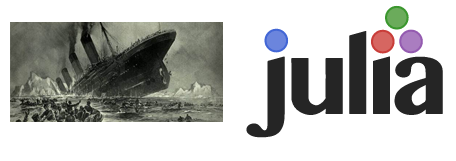# Kaggle titanic challenge with Julia commentaryKaggle titanic challenge is a famous knowledge competition which many new Kaggler will try their first Kaggle competition. Below commentary will be based on the nbviewer.

### FYI

There are also jupyter docker out there, it will be suitable if there are no GPU involved in your machine learning application.

Recently Julia is on the trend, due to its purpose of becoming an easy-to-use scripting language, while giving near to C performance speed. I always see it as combination of Python + R + C, while some might think it as Python + Matlab + C

### Commentary

using Gadfly
using DataFrames
describe(df)

• Gadfly is a popular Julia package to create the graph, equivalent to python matplotlib
• DataFrames is useful package to read and store tabular data., equivalent to python panda
typeof(df)
df[1,:]
df[:Name]

• I will use dump(df) though :)
pool!(df,[:Sex])
pool!(df,[:Survived])
pool!(df,[:Pclass])

• Using pool is to make df[:Sex], df[:Survived], df[:Pclass] to become a factor, a bit similar to a dictionary.
• By doing this, df[:Sex] will become DataArrays.PooledDataArray{UTF8String,UInt8,1} instead of DataArrays.DataArray{UTF8String,1}
plot(df,x="Sex",color="Survived",Geom.histogram)

• Generating graph, however not working in my local, seems like something is broken in Gadfly
df[!isna(df[:Age]),:]
averageAge=mean(df[!isna(df[:Age]),:Age])
df[:Age]=array(df[:Age],averageAge)

• From the describe(df), we can see that there are 177 NAs, so it is important to replace NAs data to average age
• array(da::DataArray{T}, replacement::Any) is deprecated. (as the author run this long ago)
typeof(df[:Sex])
plot(x=df[!isna(df[:Embarked]),:Embarked],Geom.histogram)
df[:Embarked]=array(df[:Embarked],utf8("S"))
pool!(df,[:Embarked])
typeof(df[:Embarked])

• Due to NAs of Embarked, one of the options is to replace NAs with the most occurence of Embarked data, based on the plot above
newdata=df[:,[:Pclass,:Age,:Sex,:SibSp,:Parch,:Fare,:Embarked]]
describe(newdata)

• The author decided to make a prediction based on the column above: Pclass, Age, Sex, SibSp, Parch, Fare, Embarked.
using DecisionTree
xTrain=newdata
yTrain=df[:Survived]
yTrain=array(yTrain)
accuracy = nfoldCV_forest(yTrain, xTrain, 5, 20, 4, 0.7)

• DecisionTree package is similar to python sklearn.ensemble.RandomForestClassifier
• Testing in my local, nfoldCV_forest has no method matching nfoldCV_forest, probably due to upgraded version of dataframe
Written on October 31, 2015